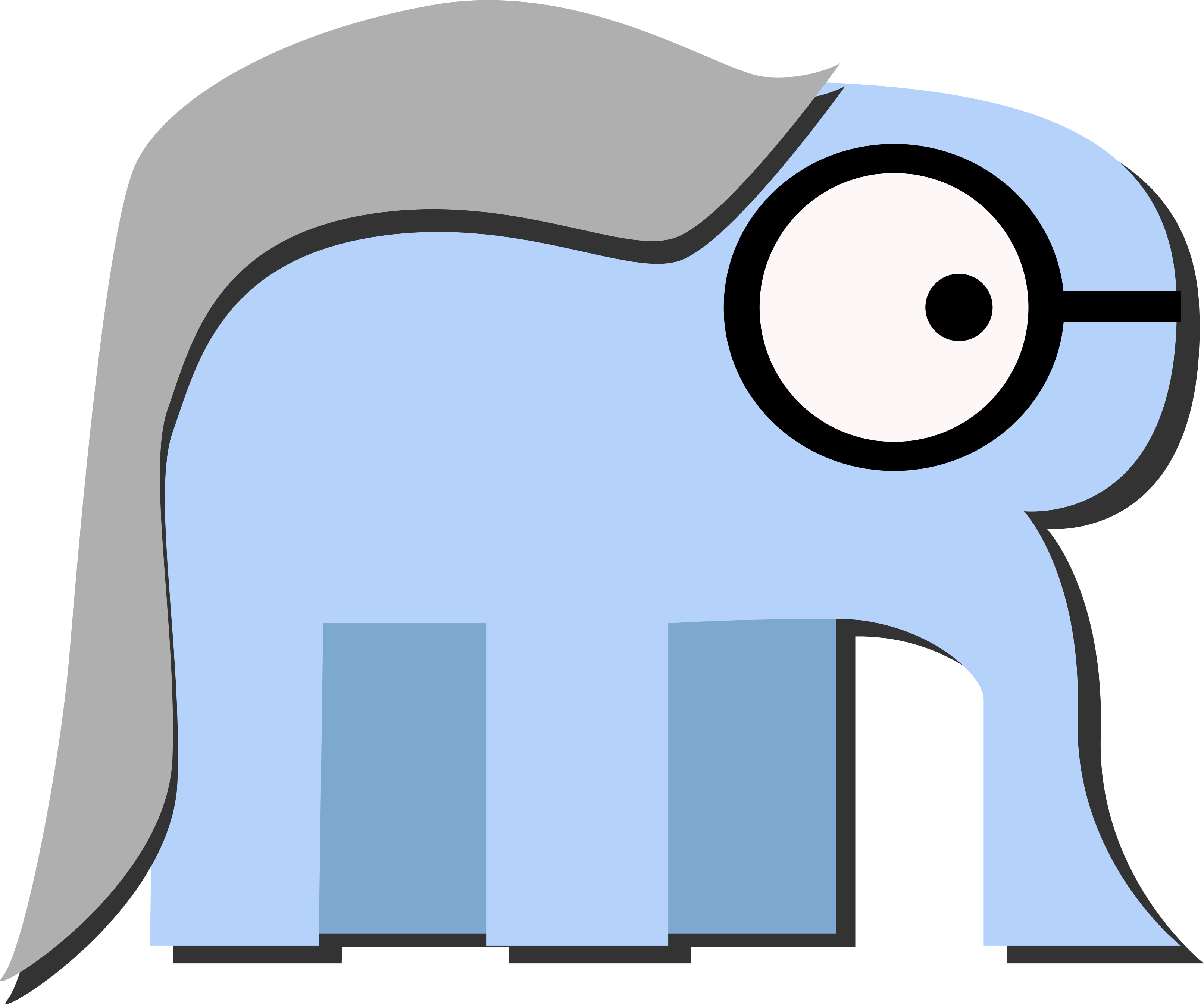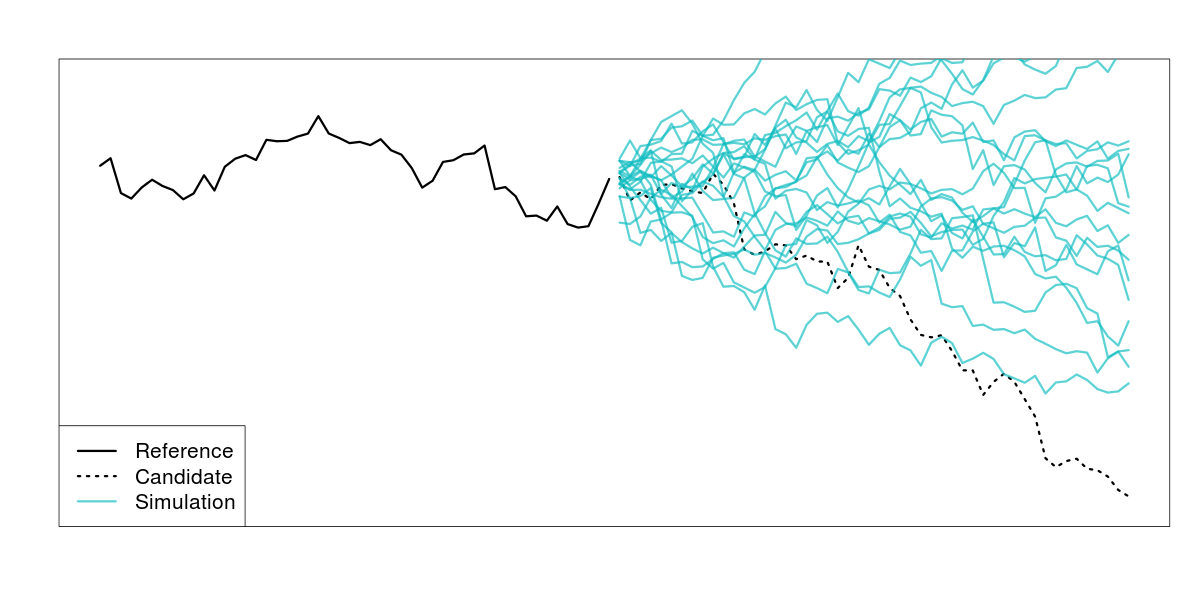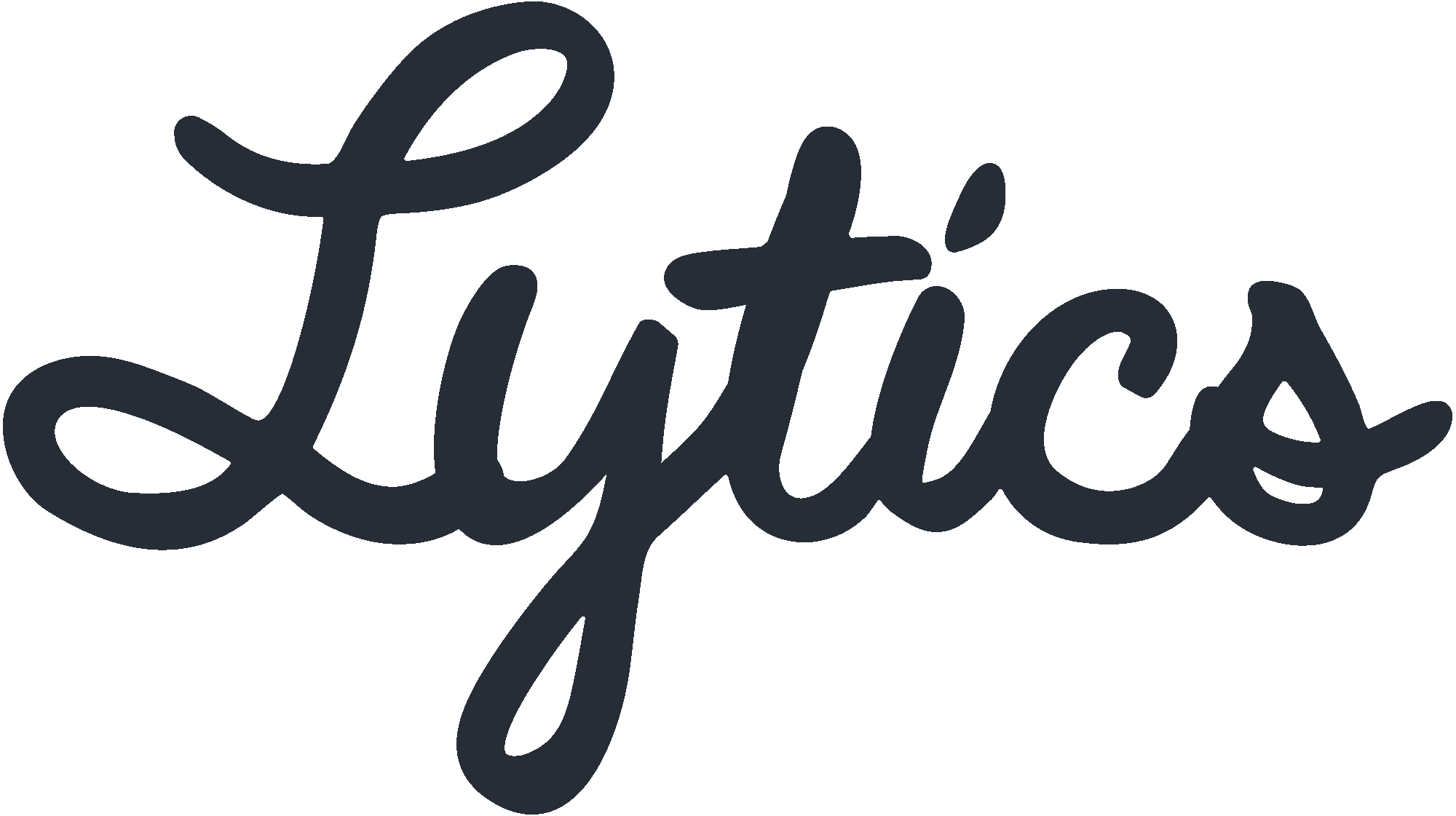# Using The Right Tool For the Job

## Prototyping in R, Productionalizing in Go

Drew Lanenga, Data Scientist, Lytics

## R + Go## Confessions

Who has ever made R do something it probably wasn't supposed to do?## Why R?

• Plots/Visualization
• Great community support, rich library
• Support from academia and industry
• Built for math/stats
• Dynamic, flexible (non-judgemental)
• Interactive/REPL/Exploration
• Fun!

## Why Not R?

• Running R in production is terrible
• Memory
• Stack Traces
• Tests/Assertions :(
• Slow (iteration, memory access)
• Poor parallelization

## Why Go?

• Great community support (Backed by Google)
• Growing libraries
• Statically typed
• Collaborative
• Readable, many syntactic decisions are made for you (`go fmt`)
• Stable/Maintainable
• Also fun!

## Why Not Go?

• Not numerically oriented
• Difficult for exploration
• No visualization
• (Initial) Development isn't as fast as R

## Hello, Go!

``````package main

import (
"fmt"
)

func main() {
fmt.Println("Hello, World!")
}
``````

## Build & Install

``````hello
|-- hello.go

\$ cd hello
\$ go build && go install  # compile, then install on \$PATH
\$ hello
Hello, World!

``````

## Functions

``````func sum(x []float64) float64 {
var total float64
for i := 0; i < len(x); i++ {
total += x[i]
}
}
``````

## Functions

``````func sum(x []float64) float64 {
var total float64
for i, value := range x {
total += value
}
}
``````

## Types

``````package vector

type Vector []float64

func (v Vector) Sum() float64 {
var sum float64
for _, value := range v {
sum += value
}
return sum
}

``````

## Tests

``````package vector

import (
"testing"
"github.com/bmizerany/assert"
)

func TestSum(t *testing.T) {
x := Vector{1.0, 2.0, 3.0}
assert.Equal(t, 6.0, x.Sum())
}

``````

## Build & Test

``````vector
|-- vector.go
|-- vector_test.go

\$ cd vector
\$ go test
PASS
ok      github.com/drewlanenga/vector   0.004s
``````

## Problem: Detecting Changes in Marketing Segments

• Lytics is a marketing data hub, handling data aggregation and resolution from many sources
• Marketers often use segments to treat different users differently
• Marketers engage users on different channels (web, email, mobile, social, POS, etc.)
• Question: Does what I'm doing make any difference?

## Segment of Dormant Users## Random Walk

• Let's consider a time series of segment sizes to be a random walk
• We want to test whether there was a change in the location of the series
• Hypothesis: Is there any way to arrive where we got if there were not a location shift?
• Test: Bootstrap a series of random walks to see if the resulting location is due to chance

## Segment of Dormant Users## Segment of Dormant Users## Segment of Repeat Purchasers## Segment of Repeat Purchasers## R Code

``````walks <- function(x1, x2, niter) {
x1.diff <- diff(x1)
destinations <- rep(0, niter)
for(i in 1:niter) {
steps <- sample(x1.diff, length(x2.smooth), replace = TRUE)
destinations[i] <- x1[length(x1)] + sum(steps)
}
return(list(
lower = mean(destinations < x2[length(x2)],
upper = mean(destinations > x2[length(x2)]
))
}
``````

## Channels/Concurrency

Do not communicate by sharing memory; instead, share memory by communicating.

• Goroutines facilitate concurrent execution
• Channels facilitate communication across goroutines

## Vector Type

``````package walker

type Vector []float64

func (v Vector) Diff() Vector {
y := make(Vector, len(v)-1)
for i := 0; i < len(y); i++ {
y[i] = v[i+1] - v[i]
}
return y
}
``````

## Randomization

``````var (
rnd   = rand.New(rand.NewSource(time.Now().UnixNano()))
rndmu = &sync.Mutex{}
)
``````
• `rnd` is not thread safe. If we're using `rnd` in separate goroutines, we'll need to lock it.

## Random Walk

``````func walk(nsteps int, steps Vector) float64 {
var dest float64
for i := 0; i < nsteps; i++ {
rndmu.Lock()
which := rnd.Intn(len(steps))
rndmu.Unlock()

dest += steps[which]
}
return dest
}
``````

## Bootstrapped Random Walk

``````func Walks(niter, nsteps int, dest float64, history Vector)
(float64, float64) {
destinations := make(Vector, niter)
steps := history.Diff()
for i := 0; i < niter; i++ {
destinations.Walk(i, nsteps, steps)
}
// do stuff to make comparisons to calculate
// p-values from destinations
return plow, phigh
}
``````

## Bootstrapped Random Walk

``````func (v Vector) Walk(i, nsteps int, steps Vector) {
dest := walk(nsteps, steps)
v[i] = dest
// no need to return anything, updated in place
}
``````

## Concurrent Bootstrapped Random Walk

``````func Walks(niter, nsteps, ncpu int, dest float64, history Vector)
(float64, float64) {
runtime.GOMAXPROCS(ncpu)
destinations := make(Vector, niter)
steps := history.Diff()
c := make(chan int, ncpu)
for i := 0; i < niter; i++ {
// launch each walk in its own goroutine
go destinations.Walk(i, nsteps, steps, c)
}
// continued on next slide
``````

## Concurrent Bootstrapped Random Walk

``````    // drain the channel
for i := 0; i < ncpu; i++ {
<-c // wait for a task to complete
}

// calculate p-values
return compare(destinations, dest)
}
``````

## Concurrent Bootstrapped Random Walk

``````func (v Vector) Walk(i, nsteps int, steps Vector, c chan int) {
dest := walk[nsteps]
v[i] = dest

c <- 1 // send anything back to notify completion
}

``````
``````func compare(destinations Vector, dest float64) (float64, float64) {
nsteps := float64(len(destinations))
var nlow, nhigh float64
for _, dest := range destinations {
if dest > realDest {
nlow += 1.0
} else if dest < realDest {
nhigh += 1.0
}
}
return nlow / nsteps, nhigh / steps
}
``````

## Go Resources